Electric Potential Energy Electric Potential AP Physics C

• Slides: 24-Electric Potential Energy -Electric Potential AP Physics C Mrs. CoyleGravitational Potential Energy Higher Potential Energy Lower Potential Energy A DUA->B= UB-UA= - Wfield BConsider a positive test charge in the following electric field. Higher Potential Energy A + DUA->B= UB-UA= - Wfield Lower Potential Energy BElectric Potential Energy When a point charge qo is in an electric field E: n The force from the field is F=qo. E n The work that the force of the electric field does to move the charge from point A to B (in the direction of the field) is W=Fd n As the charge is moved by the electric force it loses potential energy but gains kinetic energy.Change in Electric Potential Energy n n Change in Electric Potential Energy (Final PE- Initial PE) DUA->B= UB-UA= - Wfield n The (-) sign (- Wfield ) indicates the loss of potential energy when the charge moves in the direction of the field.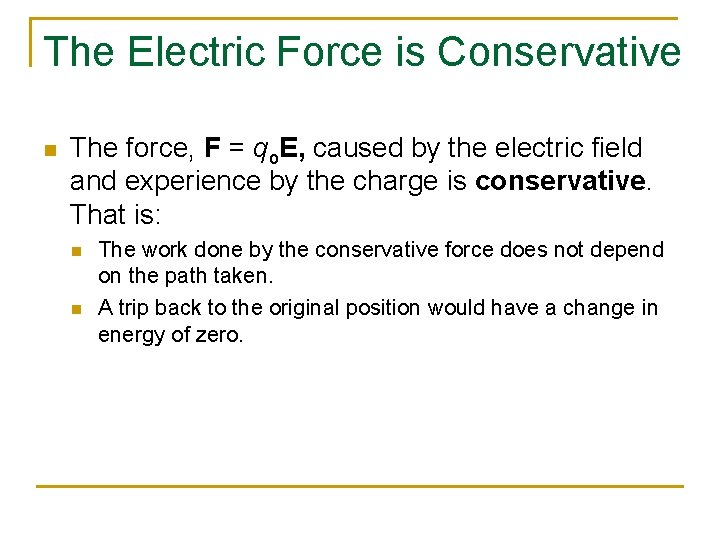The Electric Force is Conservative n The force, F = qo. E, caused by the electric field and experience by the charge is conservative. That is: n n The work done by the conservative force does not depend on the path taken. A trip back to the original position would have a change in energy of zero.n Consider an infinitesimal displacement vector , ds, that is oriented tangent to a path through space. ds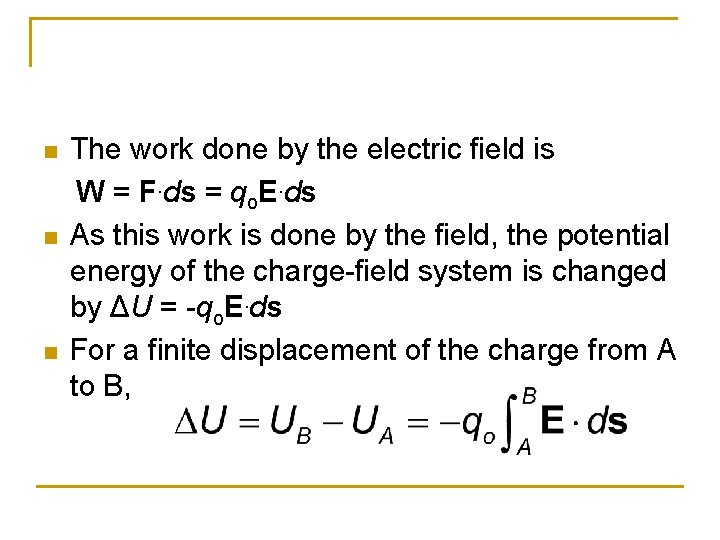n n n The work done by the electric field is W = F. ds = qo. E. ds As this work is done by the field, the potential energy of the charge-field system is changed by ΔU = -qo. E. ds For a finite displacement of the charge from A to B,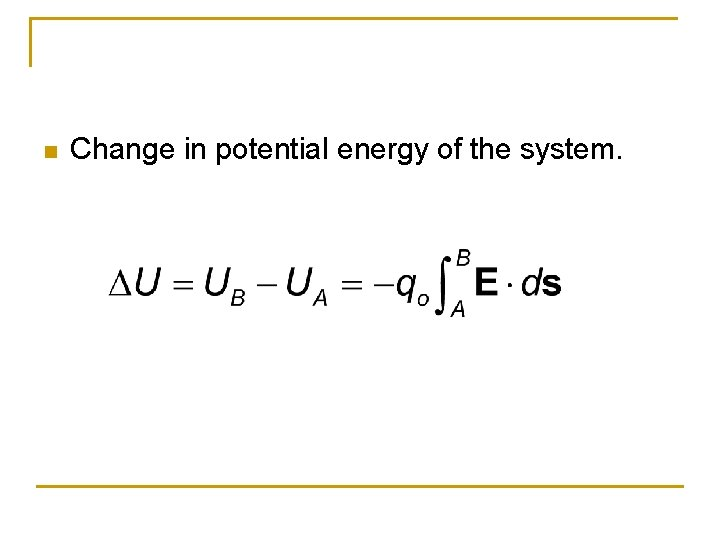n Change in potential energy of the system.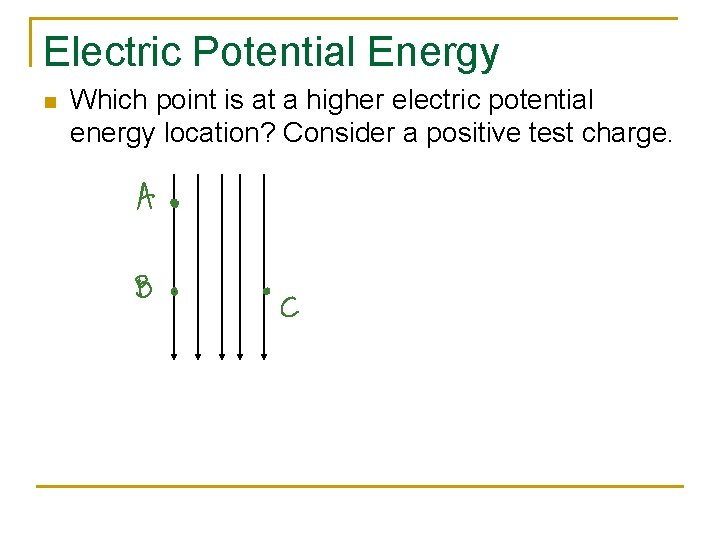Electric Potential Energy n Which point is at a higher electric potential energy location? Consider a positive test charge.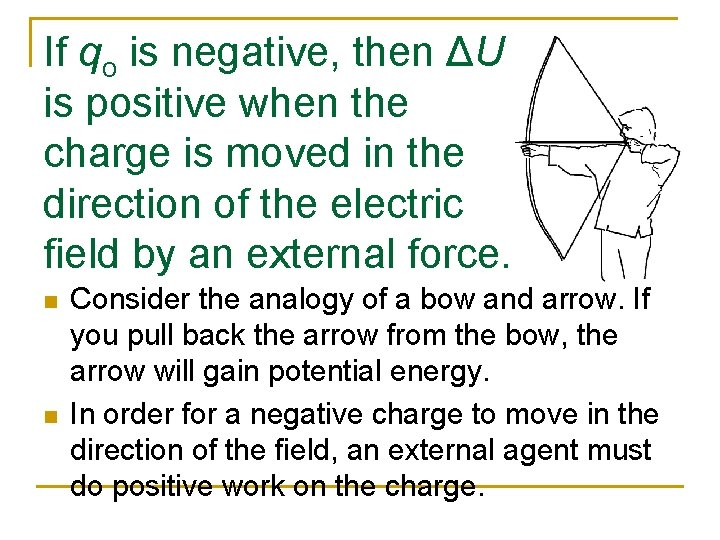If qo is negative, then ΔU is positive when the charge is moved in the direction of the electric field by an external force. n n Consider the analogy of a bow and arrow. If you pull back the arrow from the bow, the arrow will gain potential energy. In order for a negative charge to move in the direction of the field, an external agent must do positive work on the charge.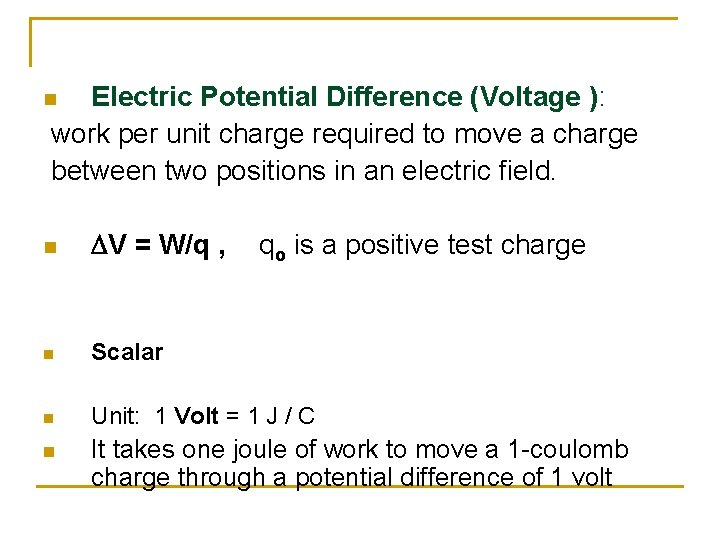Electric Potential Difference (Voltage ): work per unit charge required to move a charge between two positions in an electric field. n n DV = W/q , n Scalar n Unit: 1 Volt = 1 J / C n It takes one joule of work to move a 1 -coulomb charge through a potential difference of 1 volt qo is a positive test charge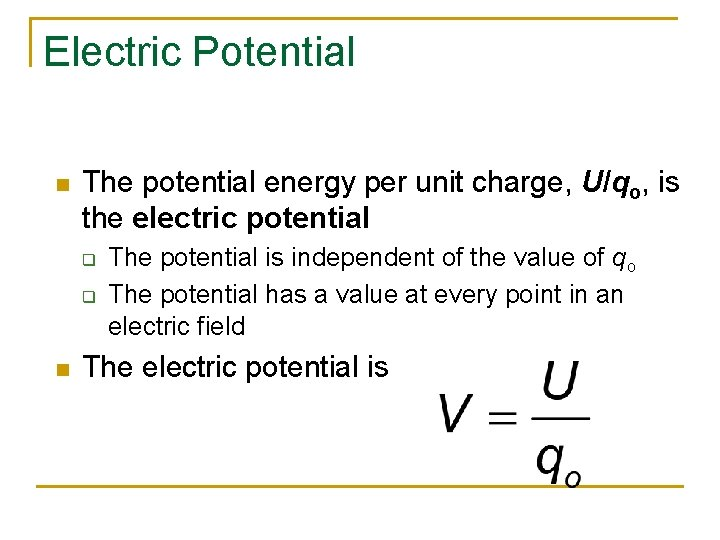Electric Potential n The potential energy per unit charge, U/qo, is the electric potential q q n The potential is independent of the value of qo The potential has a value at every point in an electric field The electric potential is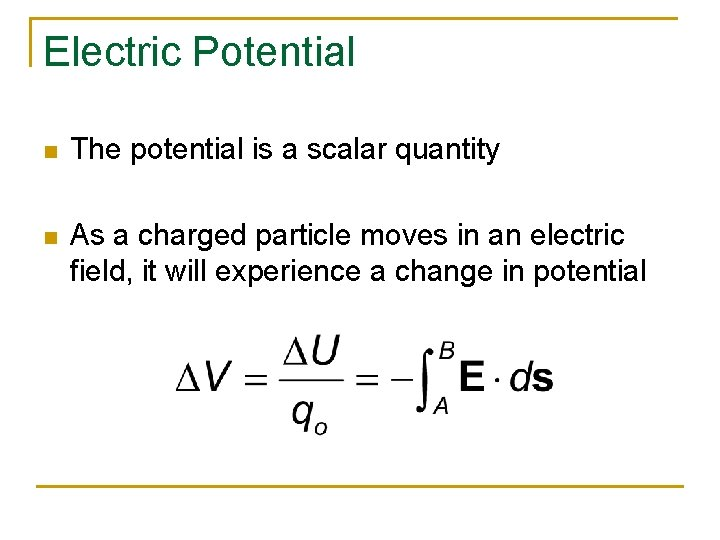Electric Potential n The potential is a scalar quantity n As a charged particle moves in an electric field, it will experience a change in potentialUsing Conservation of Energy n n Using conservation of energy, if the voltage difference is known, the potential energy difference can be calculated for a given charge. If all the potential energy is converted to kinetic energy, then final speed can be calculated.Electron-Volts n A unit of energy n One electron-volt is defined as the energy a charge-field system gains or loses when a charge of magnitude e (an electron or a proton) is moved through a potential difference of 1 volt q 1 e. V = 1. 60 x 10 -19 J q Remember that e=1. 60 x 10 -19 CSummary of Charged Particle Released from Rest in a Uniform Field n n A positive charge released from rest moves in the direction of the electric field. The change in potential is negative. The change in potential energy is negative. The force and acceleration are in the direction of the field.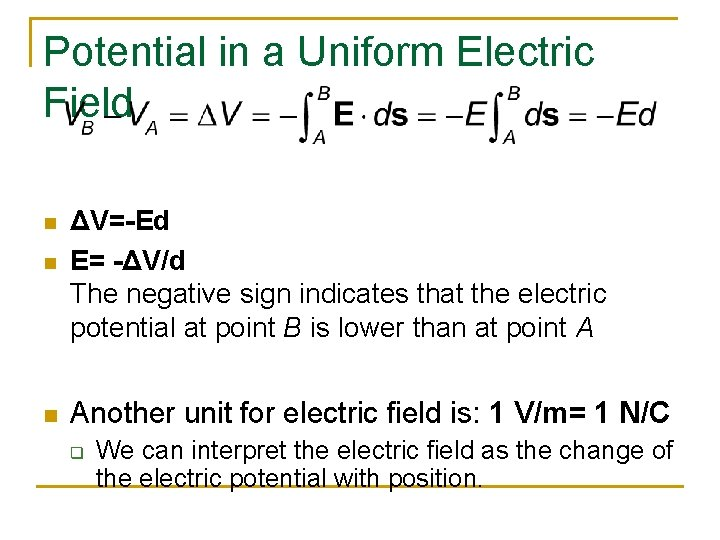Potential in a Uniform Electric Field n n n ΔV=-Ed E= -ΔV/d The negative sign indicates that the electric potential at point B is lower than at point A Another unit for electric field is: 1 V/m= 1 N/C q We can interpret the electric field as the change of the electric potential with position.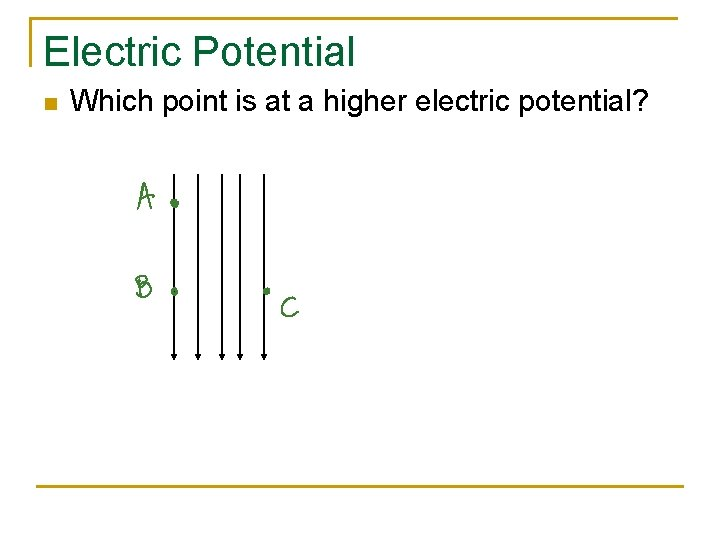Electric Potential n Which point is at a higher electric potential?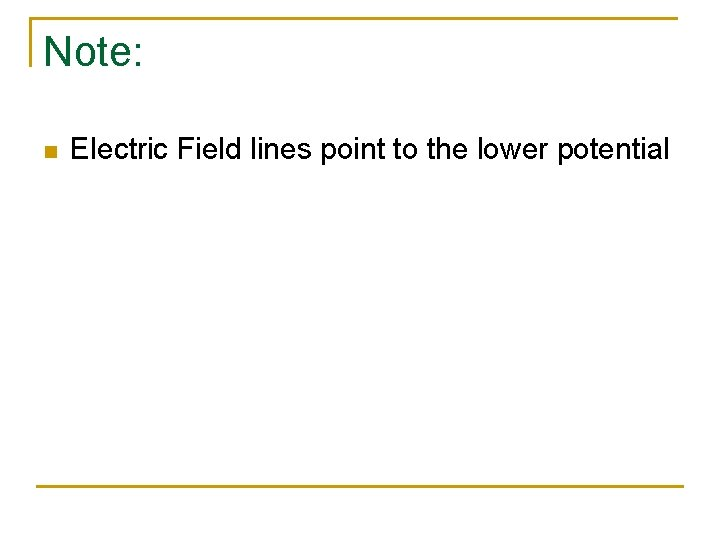Note: n Electric Field lines point to the lower potential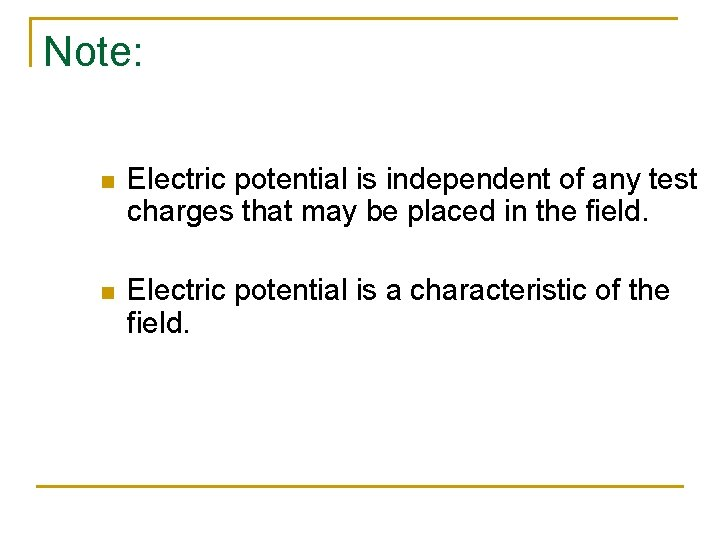Note: n Electric potential is independent of any test charges that may be placed in the field. n Electric potential is a characteristic of the field.Equipotentials n n n Point B is at a lower potential than point A Points B and C are at the same potential Equipotential surface is a surface consisting of a continuous distribution of points having the same electric potential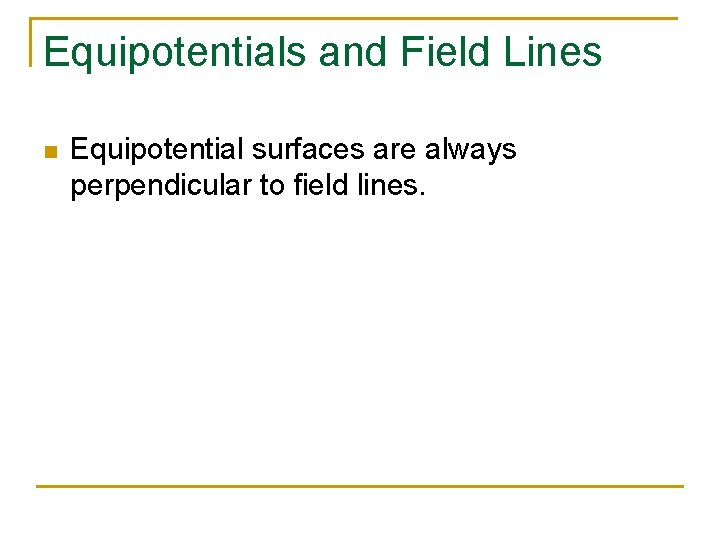Equipotentials and Field Lines n Equipotential surfaces are always perpendicular to field lines.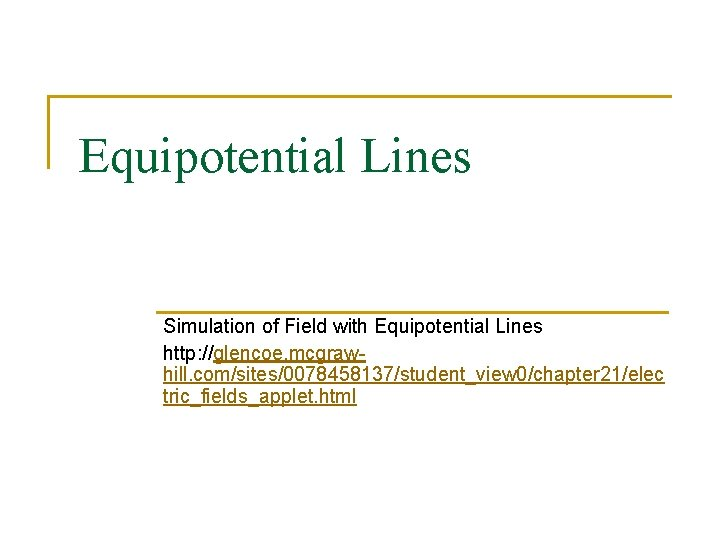Equipotential Lines Simulation of Field with Equipotential Lines http: //glencoe. mcgrawhill. com/sites/0078458137/student_view 0/chapter 21/elec tric_fields_applet. html